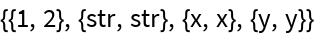# FindRanges

Contributed by: Jan Pöschko (Wolfram Research)

Find continuous ranges in a list of integers

 ResourceFunction["FindRanges"][list] returns ranges of consecutive values for the integers in list.

## Details and Options

The list is sorted before detecting ranges. The returned ranges are in ascending order.

## Examples

### Basic Examples

Find ranges of consecutive values in a list of integers:

 In:=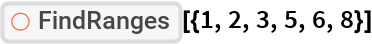Out=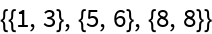### Scope

The given list does not need to be sorted, but will be sorted automatically:

 In:=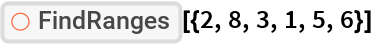Out=### Properties and Relations

The returned ranges can be joined into the original set of numbers again:

 In:=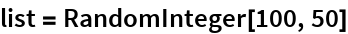Out=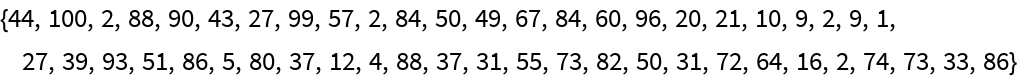In:=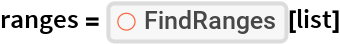Out=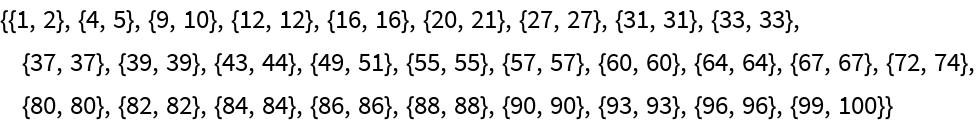In:=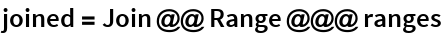Out=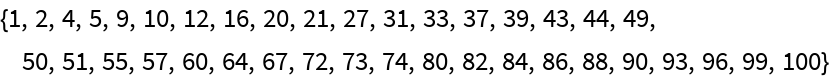In:=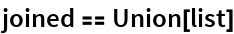Out=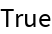### Possible Issues

Non-integer numbers are “swallowed” if they’re between consecutive integers in the list:

 In:=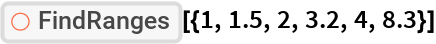Out=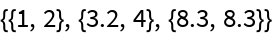Expressions that are not integers or real numbers constitute their own “range” each:

 In:=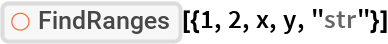Out=